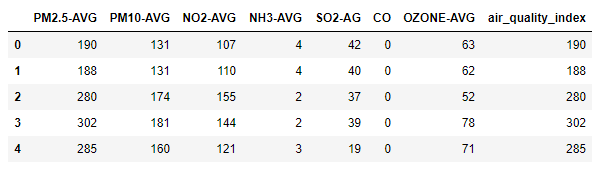Related Articles

# Predicting Air Quality Index using Python

• Difficulty Level : Medium
• Last Updated : 01 Jun, 2021

Let us see how to predict the air quality index using Python. AQI is calculated based on chemical pollutant quantity. By using machine learning, we can predict the AQI.

AQI: The air quality index is an index for reporting air quality on a daily basis.  In other words, it is a measure of how air pollution affects one’s health within a short time period. The AQI is calculated based on the average concentration of a particular pollutant measured over a standard time interval. Generally, the time interval is 24 hours for most pollutants, 8 hours for carbon monoxide and ozone.

We can see how air pollution is by looking at the AQI

Let’s find the AQI based on Chemical pollutants using Machine Learning Concept.

### Data Set Description

It contains 8 attributes, of which 7 are chemical pollution quantities and one is Air Quality Index. PM2.5-AVG, PM10-AVG, NO2-AVG, NH3-AVG, SO2-AG, OZONE-AVG are independent attributes. air_quality_index is a dependent attribute. Since air_quality_index is calculated based on the 7 attributes.

As the data is numeric and there are no missing values in the data, so no preprocessing is required. Our goal is to predict the AQI, so this task is either Classification or regression. So as our class label is continuous, regression technique is required.

Regression is supervised learning technique that fits the data in a given range. Example Regression techniques in Python:

• Random Forest Regressor
• Bagging Regressor
• Linear Regression etc.

## Python3

 `# importing pandas module for data frame``import` `pandas as pd` `# loading dataset and storing in train variable``train``=``pd.read_csv(``'AQI.csv'``)` `# display top 5 data``train.head()`

Output:## Python3

 `# importing Randomforest``from` `sklearn.ensemble ``import` `AdaBoostRegressor``from` `sklearn.ensemble ``import` `RandomForestRegressor` `# creating model``m1 ``=` `RandomForestRegressor()` `# separating class label and other attributes``train1 ``=` `train.drop([``'air_quality_index'``], axis``=``1``)``target ``=` `train[``'air_quality_index'``]` `# Fitting the model``m1.fit(train1, target)``'''RandomForestRegressor(bootstrap=True, ccp_alpha=0.0, criterion='mse',``                      ``max_depth=None, max_features='auto', max_leaf_nodes=None,``                      ``max_samples=None, min_impurity_decrease=0.0,``                      ``min_impurity_split=None, min_samples_leaf=1,``                      ``min_samples_split=2, min_weight_fraction_leaf=0.0,``                      ``n_estimators=100, n_jobs=None, oob_score=False,``                      ``random_state=None, verbose=0, warm_start=False)'''` `# calculating the score and the score is  97.96360799890066%``m1.score(train1, target) ``*` `100` `# predicting the model with other values (testing the data)``# so AQI is 123.71``m1.predict([[``123``, ``45``, ``67``, ``34``, ``5``, ``0``, ``23``]])` `# Adaboost model``# importing module` `# defining model``m2 ``=` `AdaBoostRegressor()` `# Fitting the model``m2.fit(train1, target)` `'''AdaBoostRegressor(base_estimator=None, learning_rate=1.0, loss='linear',``                  ``n_estimators=50, random_state=None)'''` `# calculating the score and the score is  96.15377360010211%``m2.score(train1, target)``*``100` `# predicting the model with other values (testing the data)``# so AQI is 94.42105263``m2.predict([[``123``, ``45``, ``67``, ``34``, ``5``, ``0``, ``23``]])`

Output:

By this, we can say that by given test data we got 123 and 95 so the AQI is Unhealthy.

Attention geek! Strengthen your foundations with the Python Programming Foundation Course and learn the basics.

To begin with, your interview preparations Enhance your Data Structures concepts with the Python DS Course. And to begin with your Machine Learning Journey, join the Machine Learning – Basic Level Course

My Personal Notes arrow_drop_up